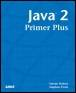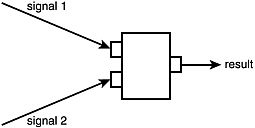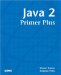# Bit-Wise OperatorsJava™ 2 Primer PlusBy Steven Haines, Steve Potts Table of Contents Chapter 3.  Operators

Remember the discussion about data types and memory from Chapter 2, "Keywords, Data Types, and Variables," in which you learned that computers think in terms of 1s and 0s, and that data types really only define the grouping and interpretation of these 1s and 0s. These 1s and 0s can be traced back to the underlying hardware implementation of computers and the principals of electrical engineering. At the very rudimentary levels of computer architecture, decisions have to be made based on two signals and whether or not they have a charge. Figure 3.1 shows this graphically.

##### Figure 3.1. Electrical engineering decision circuit.Figure 3.1 shows that a circuit makes its decision as to whether or not to send a charged signal based on the two input signals. Specific rules inside the circuit define whether the signal is charged (1) or not charged (0). These decisions can be summarized by a truth table. A truth table lists all the possible values and the required result. In the case of two signals that can be charged (1) or not charged (0), there are four possible combinations, as shown in Table 3.2.

##### Table 3.2. Truth Table Components

Signal A

Signal B

Result

0

0

1

0

0

0

1

1

1

Table 3.2 tells you that if you have no charge on Signal A (0) and a charge on signal B (1), do not send a charge (0) (second row). But if you have a charge on signal A (1) and a charge on signal B (1), send a charge (the last row).

The operators covered thus far in this chapter have been manipulating the interpreted values of these bits (for example, if a was an int with the value 10, a + 1 is equal to 11), but there are some things that can be done directly with those 1s and 0s. Java provides a set of bit-wise operators that looks at each bit in two variables, performs a comparison, and returns the result. The nature of the operator is defined by its truth table.

For the examples in this section, consider the following two bytes:

` byte a = 10;  byte b = 6; `

Recall from Chapter 2 that the bits for these values are

` a = 0000 1010  b = 0000 0110 `

The bit-wise operators are going to define rules in the form of truth tables to apply to these two values for the purpose of building a result. The general form of a bit-wise operation is

` result = operandA bit-wise-operator operandB `

The valid bit-wise operators are shown in Table 3.3.

##### Table 3.3. Bit-Wise Operators

Operator

Description

&

AND

|

`OR`

^

XOR(Exclusive OR)

~

NOT

#### AND

The AND operator (&) defines a bit-wise comparison between two variables according to the truth table shown in Table 3.4.

##### Table 3.4. AND Bit-Wise Operator

Signal A

Signal B

Result

0

0

0

0

1

0

1

0

0

1

1

1

The AND operator specifies that both Signals A and B must be charged for the result to be charged. Therefore, AND-ing the bytes 10 and 6 results in 2, as follows:

` a = 0000 1010 (10)  b = 0000 0110 (6)      ---- ---- r = 0000 0010 (2) `

#### OR

The OR operator (|) defines a bit-wise comparison between two variables, according to the truth table shown in Table 3.5.

##### Table 3.5. OR Bit-Wise Operator

Signal A

Signal B

Result

0

0

0

0

1

1

1

0

1

1

1

1

The OR operator specifies that the result is charged if either Signals A or B are charged. Therefore, OR-ing the bytes 10 and 6 results in 14, as follows:

` a = 0000 1010 (10)  b = 0000 0110 (6)      ---- ---- r = 0000 1110 (14) `

#### Exclusive OR

The Exclusive OR (XOR) operator (^) defines a bit-wise comparison between two variables according to the truth table shown in Table 3.6.

##### Table 3.6. XOR Bit-Wise Operator

Signal A

Signal B

Result

0

0

0

0

1

1

1

0

1

1

1

0

The XOR operator specifies that the result is charged if Signal A or B is charged, but Signal A and B aren't both charged. It is called exclusive because it is charged only if one of the two is charged. Therefore, XOR-ing the bytes 10 and 6 results in 12, as follows:

` a = 0000 1010 (10)  b = 0000 0110 (6)      ---- ---- r = 0000 1100 (12) `

#### NOT Operator

The NOT operator (~), also referred to as the bit-wise complement, flips the values of all the charges. If a bit is set to 1, it changes it to 0; if a bit is 0 it changes to 1.

#### Practical Application

At this point you are probably telling yourself that this is a neat thing you can do, but asking yourself the inevitable question: "Who cares!?"

The primary use for bit-wise operations originated in the data compression and communication applications. The problem was that you needed to either store or transmit the state of several different things. In the past, data storage was not as cheap as it is today and communication mechanisms were not over T3 or even a cable modem, but more like speeds of 1200 or 300 baud. To give you an idea about the difference in speed, cable modem and DSL companies tout that they achieve speeds about 20 times faster than 56K modems, but 56K modems are about 45 times faster than 1200-baud modems, and 180 times faster than 300-baud modems. So early communications were 3600 times slower than your cable modem. Thus, there was the need to compact data as much as possible!

If you have 8 different states to send to someone, you can simply assign a bit in a byte to each of the 8 states: 1 is defined to be true and 0 false (or on/off, and so on). Consider reporting the state of 8 different factory devices where devices 0 and 3 are active:

` Device byte: 0000 1001 (9) `

To determine whether device 3 is active, a Boolean expression can be determined with the following statement:

` boolean is3Active = ( deviceByte & 8 ) == 8; `

AND-ing the deviceByte (0000 1001) with the number 8 (0000 1000) returns 8, as the first bit is 0 (1 & 0 = 0), and using the equality operator it can be compared to 8 to see if that bit is set.

You should understand how bit-wise operators work (they are covered in Java certification exams) and, depending on what area of Java programming you delve into, you might use them in the future.TopJava 2 Primer Plus
ISBN: 0672324156
EAN: 2147483647
Year: 2001
Pages: 332

Similar book on Amazon